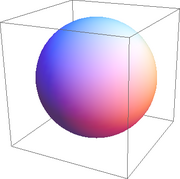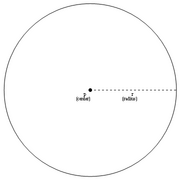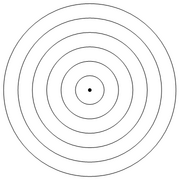# File list

23:43, 29 July 2011 2sphere.png (file)27 KB This file was created using [http://mathematica.wolfram.com Wolfram Mathematica 8.0] with the following code: <pre>Graphics3D[Sphere[{0, 0, 0}, 1]]</pre> 1
23:21, 29 July 2011 Circle.png (file)8 KB This file was created using [http://mathematica.wolfram.com Wolfram Mathematica 8] with the following code: <pre>Graphics[{Circle[{0, 0}, 1], PointSize[0.02], Point[{0, 0}], Dashed, Line[{{0, 0}, {1, 0}}], Black, Text["p", {-0.05, -0.05}], Text["(c 1
23:14, 29 July 2011 Concentriccircles.png (file)17 KB This file was created using [http://mathematica.wolfram.com Wolfram Mathematica 8.0] with the following code: <pre>Graphics[{Circle[{0, 0}, 0.5], Circle[{0, 0}, 1], Circle[{0, 0}, 1.5], Circle[{0, 0}, 2], Circle[{0, 0}, 2.5], Circle[{0, 0}, 3], Poi 1
17:03, 11 September 2008 Logo.jpg (file)32 KB   1